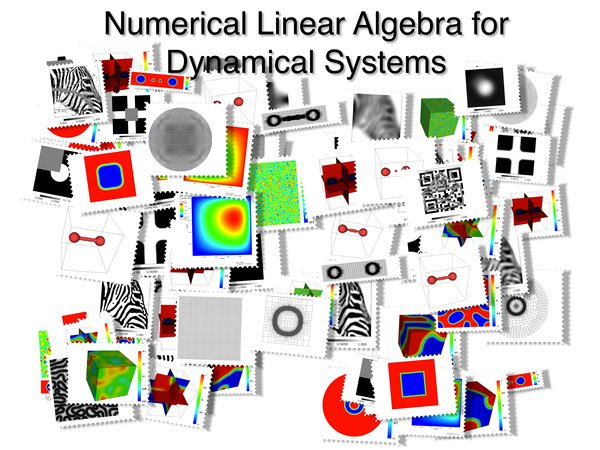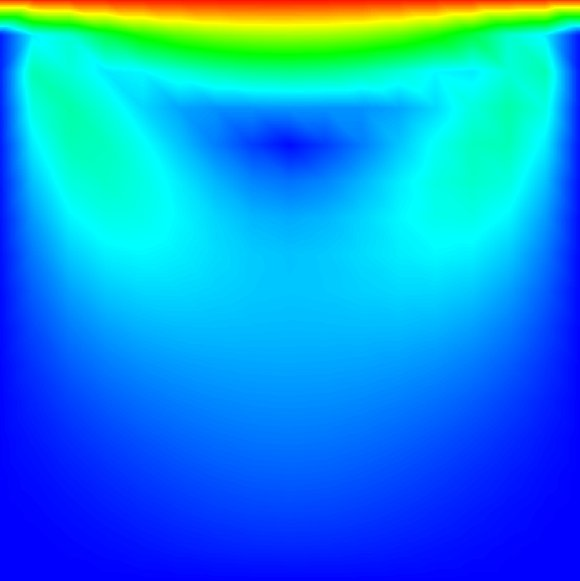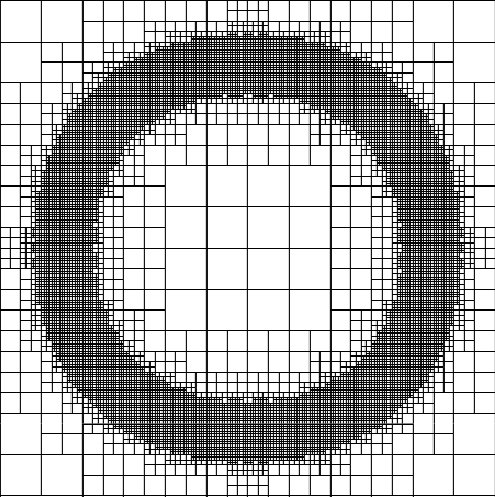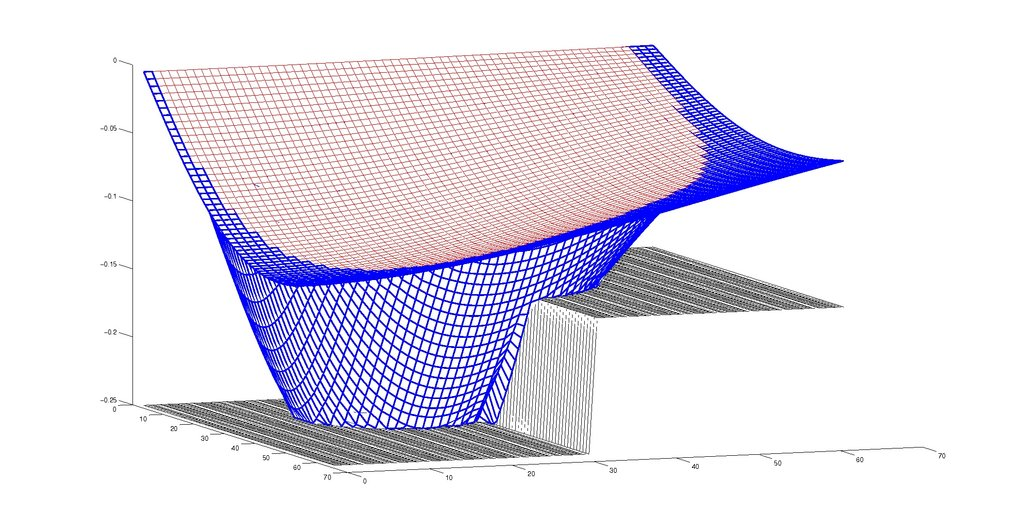## Research Numerical Linear Algebra for Dynamical Systems# Research Topics

Many processes in engineering as well as in the natural and life sciences are described by time-dependent differential equations. Our goal is to provide efficient and robust numerical solvers that enable the accurate solution of the discretized equations.### Low-rank Solver

Many of the discretized problems have an inherent tensor-structure that allows us to employ low-rank methods. We here develop state of the art techniques that enable an efficient treatment of such problems. [more]### Iterative solvers for nonlocal equations

Employing a monolithic approach representing "all of the physics" and "all-of-the optimization" in one system, we often require many time-steps and are led to large-scale problems. We here combine modern HPC techniques with state-of-the-art algorithms. [more]### Phase field solver

Many problems in material science, image processing or chemistry are well represented using phase-field equations. We here investigate schemes that enable the fast solution by also being robust to parameter changes. [more]### Preconditioning

Processes across the sciences are described by differential equations and we here consider their discretized counterparts and provide accelrated methods for obtaining an accurate solution to such problems. [more]Go to Editor View